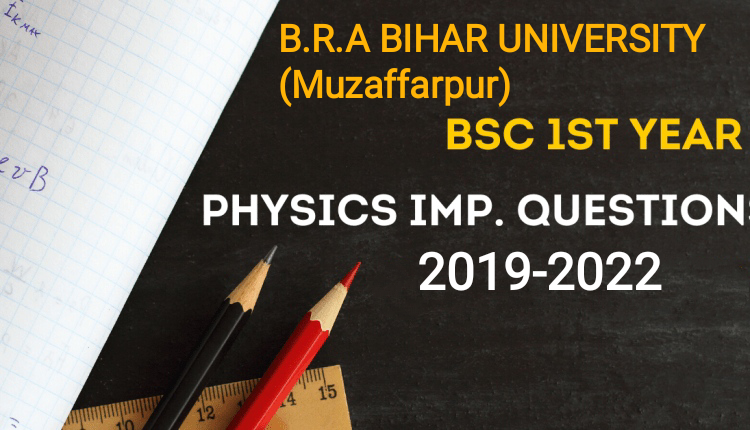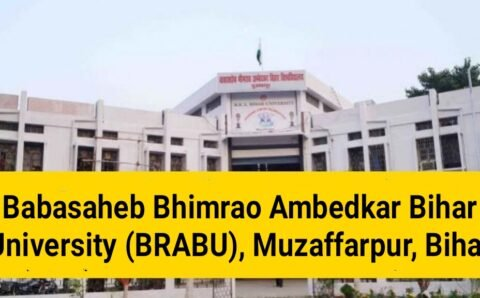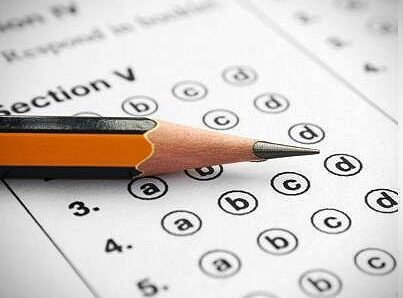# B.R.A BIHAR UNIVERSITY(Muzaffarpur) Exam Important QuestionsExam Important Questions

(DAY 1) 08-03-2021

Graduation TDC Part 1 (2019-2022) Math/Physics/Chemistry

PG 1st semester (2019-21) – Physics / Math – Important questions

Are you searching for BSc 1st year and PG 1st semester important questions in Physics/Math/Chemistry? Well, you are in the right place. In this post, we are go to share some of the most important questions of Physics/Math/Chemistry for TDC Part 1and PG 1st semester students. (B.R.A BIHAR UNIVERSITY)

(Day 1)

(08-03-2021) TDC PART 1 PHYSICS (Hons.)

PHYSICS (Hons.) Paper-1

TDC Part 1 (2019-22)

(Answer any six questions, selecting at least one from Group A, four from Group B and one from Group-C.)

## Group A

1. Obtain an expression for mass-energy equivalence and discuss its physical significances.
2. Derive the formula for the variation of mass of a particle with its velocity. Find the velocity of the particle at which the mass of the particle becomes double of its rest mass.
3. Discuss critically the postulates of special theory of relativity. Derive Lorentz transformation equations.
4. Describe Michelson-Morley experiment and explain the significance of negative result.

## Group-B

1. State and explain Kepler’s laws of planetary motion and deduce the laws, Define gravitational potential and field intensity and obtain their relation. Obtain gravitational potential and intensity at a point due to a uniform solid sphere of mass M and radius R at distance r from its centre for r < R.
2. State and explain D’Alembert’s Principle. Use it to obtain Lagrangian equation of motion.

3. What is flat spiral spring?
4. Define the elastic constants and establish the relations between them.
5. Describe with theory, the experiment to determine surface tension of water by the method of ripples.

6. Give a critical analysis of vertical oscillations of a flat spiral spring under p a load W and obtain an expression for its time period.
7. Discuss the characteristics of stremline motion of a liquid. Establish Euler’s equation for a perfect fluid.
8. Deduce equation of continuity for a flowing fluid and discuss its application.
9. What is Hamiltonian for a system? Deduce Hamilton’s equations of motion for conservative system and discuss the physical significance of Hamiltonian.

10. Uniform beam is supported symmetrically at its ends. Obtain an expression for depression of its middle point when a load W is suspended from it.

11. Define surface tension and surface energy. State and explain the principle of virtual work.
12. Deduce an expression for the pressure difference across a curved liquid membrane
13. Describe in detail the method of determination of coefficient of viscosity Of a gas.
14. What is an inertial frame of reference? Is the earth such a frame of reference? Give reasons.
15. Discuss the concept of centrifugal force and explain the variation of the acceleration due to gravity with latitude.
16. Write short notes on any two of the following : (a) Generalized Co-ordinater (b) D’ Alemberte’s principle (c) Effect of temperature on surface tension of a liquid (d) Bernouille’s theorem .
17. Define intensity of sound and give its units. Describe an experiment for determination of intensity of sound.
18. What is reverbratioreverbratioreverbratioreverbratiom

## Group-C

1. Define intensity of sound and give its units. Describe an experiment for determination of intensity of sound.
2. Explain the term critical velocity in fluid dynamics. Obtain Poiseuille formula with correction for determination of viscosity of liquid.
3. What is reverbration?

4. Write short notes on any two of the followings (a) Holonomic and non-holonomic constraints (b) Bending of beam (c) Eqüation of continuity (d) Physical significance of Hamiltonian
5. Write an essay on Acoustics of Building.
6. Derive equations of progressive and stationary waves and discuss their characteristics.

THE END

Rn college Telegram group – Click here

Bihar University & Rn college info – Click here### स्नातक पार्ट-वन की परीक्षा के लिए क्वेश्चन बैंक बनाने में छूट रहे पसीने, हरेक विभाग को बनाना है 900 सवाल

बीआरए बिहार विश्वविद्यालय में स्नातक पार्ट-वन की परीक्षा के लिए क्वेश्चन बैंक तैयार करने में पीजी विभागों के पसीने छूट रहे हैं। हर विभाग को...### स्नातक पार्ट वन के परीक्षार्थियों को अगले माह मिलेगा मॉडल क्वेश्चन बैंक, परीक्षा के लिए मिल जाएगी अनुमति### BIHAR UNIVERSITY (Muzaffarpur)Zoology Exam Important Questions